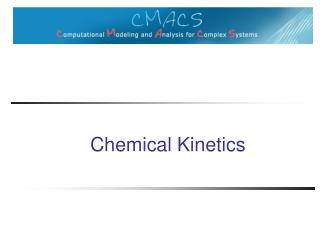DownloadDownload PresentationChemical Kinetics

# Chemical Kinetics

Download Presentation## Chemical Kinetics

- - - - - - - - - - - - - - - - - - - - - - - - - - - E N D - - - - - - - - - - - - - - - - - - - - - - - - - - -
##### Presentation Transcript

1. Chemical Kinetics

2. What is chemical kinetics • The study of the rates at which chemical reactions take place • Factors to consider • Concentration • Temperature • Presence of catalysts

3. Reaction rates The rate of a chemical reaction is a measure of how the concentration (or pressure) of the involved substances changes with time.

4. Chemical Reactions • 2Na + Cl2 2 NaC • table salt • S + O2SO2 • sulfur dioxide • 4 Fe + 3 O2 2 Fe2O3 • iron rusting • CO2 + H2OH2CO3 • carbon dioxide dissolving and reacting with water to form carbonic acid

5. Biochemical Reactions • Aerobic Respiration • Glucose+Oxygen  CarbonDioxide+Water +Energy • C6H12O6+ 6O2 6CO2 + 6H2O + energy • Anaerobic Respiration • C6H12O6 2C2H5OH+2CO2 + Energy • Glucose  Ethanol+CarbonDioxide+Energy • Metabolism of ethanol • Ethanol  Acetaldehyde • CH3CH2OH + NAD+ CH3CH=O + NADH + H+

6. Rates Part I: The Law of Mass Action .039/sec Rate: CO2 + H2O H2CO3 Reaction: Define: [A] = concentration of A (e.g., moles per liter) d[H2CO3] = k+[CO2][H2O] dt = .039[CO2][H2O]

7. The Law of Mass Action d[H2CO3] = k+[CO2][H2O] - k-[H2CO3] dt = .039[CO2][H2O] - 23[H2CO3] .039/sec Forward Rate: CO2 + H2O H2CO3  Reaction: 23/sec Backward Rate:

8. Reaction Equilibrium At equilibrium, the instantaneous forward rate equals the instantaneous reverse rate. k+[CO2][H2O] - k-[H2CO3] = 0 [CO2][H2O] k- [H2CO3] k+ K = 23/.039 ~ 590 = = K Since the equilibrium constant is large, we can predict that very little carbonic acid forms.

9. Deriving Equilibrium Values [H2O]eq K + [H2O]eq K K + [H2O]eq In the absence of other reactions involving CO2 and H2CO3, the quantity [CO2]+[H2CO3] = A0 is conserved. We can use this to compute various things: [H2CO3]eq = A0 [CO2]eq = A0

10. Forming Dimers M is a monomer D is a dimer M + M  D k+ k-  d[M] dt = 2k-[D] - 2k+[M][M]

11. Forming Dimers - Exercise d[M] dt = 2k-[D] - 2k+[M]2 d[D] dt = -k-[D] + k+[M]2 d[M] dt d[D] dt = -1/2 [M] + 2[D] is conserved

12. Organic Chemical ReactionsPhosphorylation Example of enzymatic action

13. Enzyme Kinetics • A kinase is an enzyme • Enzymes are catalysts that make reactions faster • Catalytic power • Specificity • Regulation • Can increase speeds up to 1 million times

14. Enzyme Kinetics • Enzymes don’t follow the law of mass action directly • By the law of mass action, the rate of S+E->P+Ewould increase linearly with increasing [S] • In enzymatic reactions, the rate increases less than linearly with the increase in S and reaches a maximum

15. Rate Laws: Enzymatic Action Instead of S+EP+E the reaction creates a complex SE from the substrate and enzyme, followed by dissociation of the complex into the product and the enzyme.

16. Enzymatic Action • Assumption 1: The enzyme forms a complex with the substrate, from which the product is formed. • Exercise: What is the chemical formula? k1 k2 E+S ES E+P k-2 k-1

17. Enzymatic Action • Assumption 2: The product doesn’t react with the enzyme. • Exercise: How does this change the formula? k1 k2 (k-2 = 0) E+S ES E+P k-1

18. Rate Laws: Enzymatic Action Lets=[S] (substrate), e=[E] (enzyme), c=[ES] (complex), p=[P] (product

19. Exercises ds dt = -k1se + k-1c de dt = -k1se + k2c + k-1c • Rate of change of substrate: • Rate of change of complex: • Rate of change of enzyme: • Rate of change of product: dc dt = k1se - k-1c - k2c dp dt = k2c

20. Quasi-steady State Approximation • Assumption 3: The concentration of the complex changes much more slowly than the concentrations of the substrate and the product • Exercise: How would you model this? dc dt = k1se - k-1c - k2c ≈ 0

21. Quasi-steady State Approximation • Assumption 4: The enzyme concentration doesn’t change over time. • Exercise: How would you model this?e0 = e + c is a constant

22. Exercise dp dt dc dt = k1se - k-1c - k2c ≈ 0 Using e0 = e + c is a constantand compute (the rate of change of the concentration of the product) as a function of s (the concentration of the substrate).

23. Answer to Exercise dp dt s = k2 e0 + s k2 + k-1 k1 Let KM = and vmax = k2 e0 k2 + k-1 k1 dp dt Then = vmax s KM + s

24. Consequences • What can we say about the reaction if: • s << KM? • s ≈ KM? • s >> KM? • Hint: Look at s KM + s